## Robert Harlander

 fax: +49-241-80-22187 harlander(at)physik.rwth-aachen.de phone: +49-241-80-27045

Home

Brief CV

– Research –

Teaching

Outreach

Conferences

Software

Press

Search Portal

external:

Higgs WG

LHC and Philosophy

Robert Harlander: Research Interests -/- Home -/- TTP Karlsruhe -/- CERN Theory -/- HET Brookhaven

#### Research interests: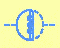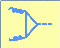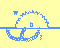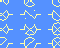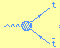### Quark mass effects in higher order QCD - Asymptotic Expansions

#### Asymptotic expansions

A central part of my Ph.D. thesis was concerned with the precise determination of the influence of finite quark masses to the hadronic cross section at electron-positron colliders. A powerful tool for this kind of calculations is provided by the method of asymptotic expansion, an effective re-formulation of the operator product expansion (for a review see ). Previously, the application of this procedure was restricted to lower orders of perturbation theory because it requires the evaluation of the huge number of diagrams generated by this approach. Therefore we automated the method which allowed to compute the third order QCD corrections to the hadronic R ratio as an expansion in the quark mass ,  (for a review on the automatic computation of Feynman diagrams, see ).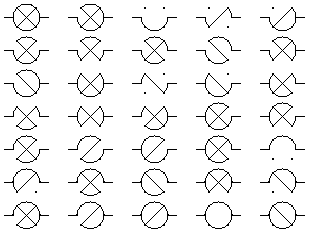Subdiagrams of the non-planar three-loop diagram that contribute to the large momentum procedure (taken from ).

#### Top quark production at a Linear Collider

It turned out that using the same strategy one could also examine top quark pair production at a linear collider. If the energy is slightly above the threshold region (click here for a treatment of the threshold region itself), the top quark mass still influences the cross section significantly. A detailed investigation of the corresponding production cross section was performed in .

#### Higgs boson decay

Also the decay of (heavy) scalar or pseudo-scalar Higgs bosons into top quarks could be investigated. Although the current limits on a Standard Model Higgs mass rule out this decay, the existence of other, heavier scalars or pseudo-scalar particles as predicted, for example, in supersymmetric theories is well possible. In a certain mass range, top quark mass effects may have a significant influence on the decay rate. This dependence was studied in  with the help of asymptotic expansions and the tools developed at the first stage of my thesis.

#### Padé approximations (for singlet diagrams)

(For a review on Padé approximations see here.) The results obtained through asymptotic expansions can be combined so that the corresponding cross sections or decay rates will be valid over the full energy range. This strategy was already known for non-singlet contributions, but in order to correctly account for axial-vector couplings one needs its generalization to singlet diagrams.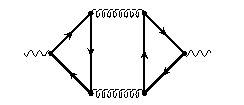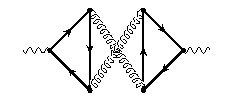"Singlet" or "double triangle" diagrams.

Furthermore, the original approach was tailored to include only the leading mass corrections, and it was important to respect the previously mentioned higher order mass terms. Both the extension to singlet diagrams and the inclusion of higher mass terms was performed in , so that now, for example, the hadronic R ratio is known completely up to order \alpha_s^2, including its axial vector part.

#### Quartic mass terms at alpha_s^3

All the discussion above is concerned with mass corrections at order alpha_s^2. In the massless case, most of the quantities above are known up to order alpha_s^3. To obtain an estimate on the mass effects at this order, the above strategy of asymptotic expansion leads to four-loop diagrams that can not be solved in general. However, using renormalization group methods, one can derive the quadratic mass terms at order alpha_s^3 from the ones at alpha_s^2. If one aims for the quartic mass terms, one may follow a similar strategy. Operator product expansion in combination with renormalization group methods allows to reduce the four-loop diagrams to three-loop ones. The method is briefly described in , while the results are presented in .

### Literature:

  R.V. Harlander, M. Steinhauser rhad: a program for the evaluation of the hadronic R-ratio in the perturbative regime of QCD , Comp. Phys. Comm. 153 (2003) 244-274. [hep-ph/0212294] [Journal Version] (the program is available from http://www.rhad.de/)  R. Harlander, Asymptotic Expansions - Methods and Applications, Acta Phys. Pol. B30 (1999) 3443-3462. (Pedagogical overview, relation among different approaches.)  K.G. Chetyrkin, R. Harlander, J.H. Kühn, M. Steinhauser Mass Corrections to the Vector Current Correlator, Nucl. Phys. B503 (1997) 339-353. (Expansion of Rhad in terms of m2/s.)  K.G. Chetyrkin, R. Harlander, J.H. Kühn, M. Steinhauser, Automatic Computation Of Three-Loop Two-Point Functions In Large Momentum Expansion, Nucl. Instrum. Meth. A389 (1997) 354-356. (Describes the technical realization of the method used in .)  R. Harlander, M. Steinhauser, Automatic Computation of Feynman Diagrams, Prog. Part. Nucl. Phys. 43 (1999) 167-228. (General overview of methods and realizations for calculating Feynman diagrams on a computer.)  R. Harlander, M. Steinhauser, O(\alpha_s^2) Corrections to Top Quark Production at e^+e^- Colliders, Eur. Phys. J. C2 (1998) 151-158. (Expansion of the total cross section in terms of mtop /sqrt(s).)  R. Harlander, M. Steinhauser, Higgs Decay to Top Quarks at O(\alpha_s^2),, Phys. Rev. D56 (1997) 3980-3990. (Expansion of the decay rate for scalar and pseudo-scalar Higgs in terms of mtop /MHiggs.)  K.G. Chetyrkin, R. Harlander, M. Steinhauser, Singlet Polarization Functions at O(\alpha_s^2),, Phys. Rev. D58 (1998) 014012. (Combines asymptotic expansion and Padé approximation.)  K.G. Chetyrkin, R.V. Harlander, J.H. Kühn, Quark mass effects to sigma(e+ e- ---> hadrons) at O(alpha_s^3) , (Proceedings of EPS-HEP 99, Finland) hep-ph/9910345 (Describes the techniques used in .)  K.G. Chetyrkin, R.V. Harlander, J.H. Kühn, Quartic mass corrections to Rhad at O(\alpha_s^3), , Nucl. Phys. B586 (2000) 56. (Uses OPE and RG techniques.)

Robert Harlander: Research Interests -/- Home -/- TTP Karlsruhe -/- CERN Theory -/- HET Brookhaven